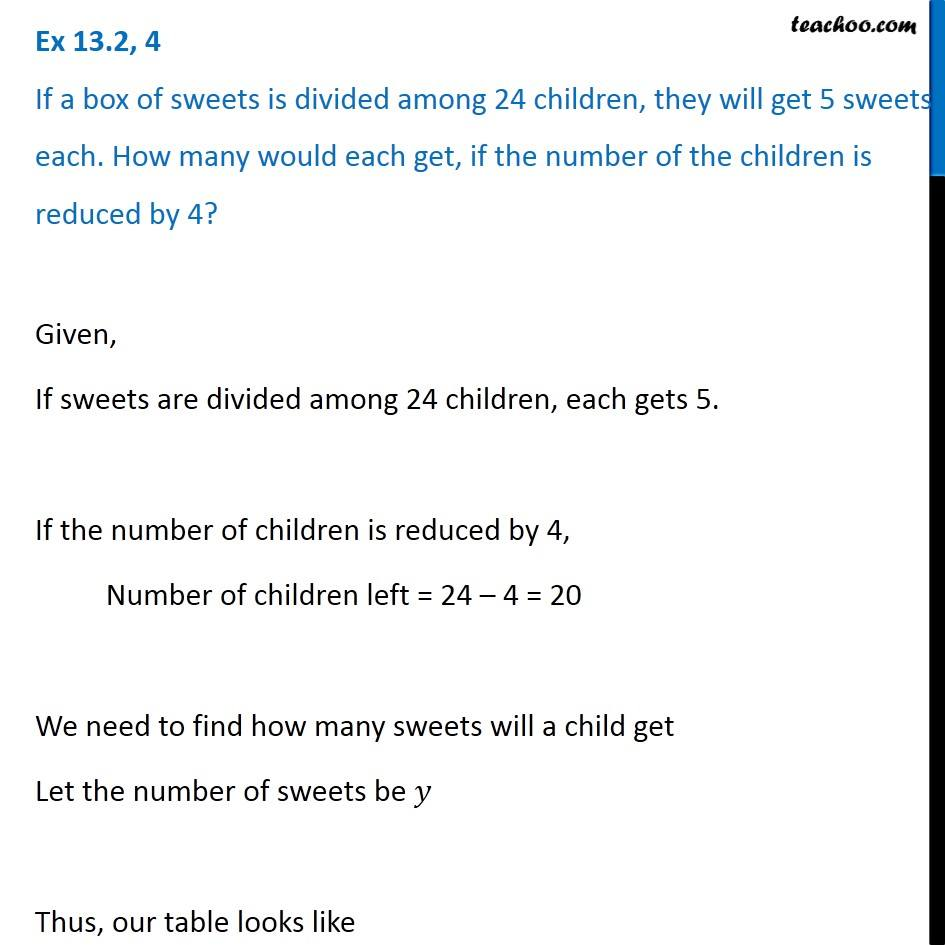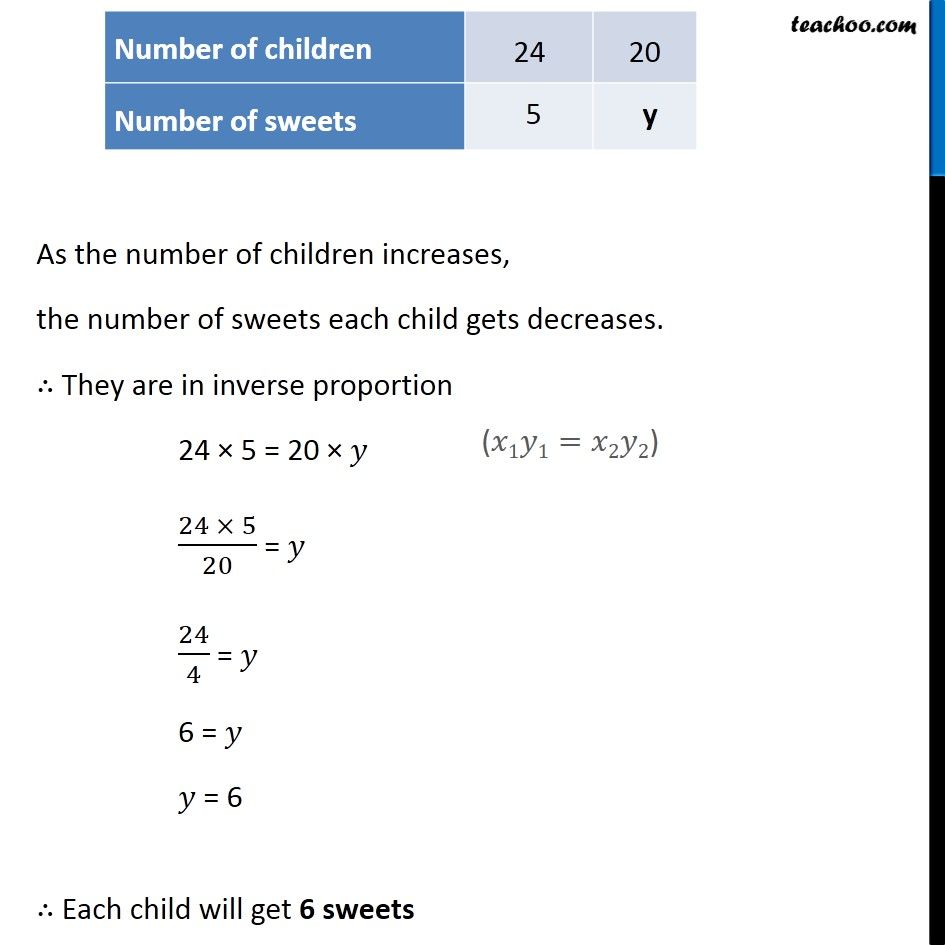Inverse Proportion

Chapter 11 Class 8 Direct and Inverse Proportions
Concept wiseLearn in your speed, with individual attention - Teachoo Maths 1-on-1 Class

### Transcript

Ex 11.2, 4 If a box of sweets is divided among 24 children, they will get 5 sweets each. How many would each get, if the number of the children is reduced by 4? Given, If sweets are divided among 24 children, each gets 5. If the number of children is reduced by 4, Number of children left = 24 – 4 = 20 We need to find how many sweets will a child get Let the number of sweets be 𝑦 Thus, our table looks like As the number of children increases, the number of sweets each child gets decreases. ∴ They are in inverse proportion 24 × 5 = 20 × 𝑦 (24 × 5)/20 = 𝑦 24/4 = 𝑦 6 = 𝑦 𝑦 = 6 ∴ Each child will get 6 sweets